Chemical Kinetics

# Chemical Kinetics

Test Description

## 20 Questions MCQ Test GATE Chemistry Mock Test Series | Chemical Kinetics

Chemical Kinetics for Chemistry 2022 is part of GATE Chemistry Mock Test Series preparation. The Chemical Kinetics questions and answers have been prepared according to the Chemistry exam syllabus.The Chemical Kinetics MCQs are made for Chemistry 2022 Exam. Find important definitions, questions, notes, meanings, examples, exercises, MCQs and online tests for Chemical Kinetics below.
Solutions of Chemical Kinetics questions in English are available as part of our GATE Chemistry Mock Test Series for Chemistry & Chemical Kinetics solutions in Hindi for GATE Chemistry Mock Test Series course. Download more important topics, notes, lectures and mock test series for Chemistry Exam by signing up for free. Attempt Chemical Kinetics | 20 questions in 60 minutes | Mock test for Chemistry preparation | Free important questions MCQ to study GATE Chemistry Mock Test Series for Chemistry Exam | Download free PDF with solutions
 1 Crore+ students have signed up on EduRev. Have you?
Chemical Kinetics - Question 1

### If A reacts to form either B or C according to reactions,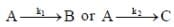if E1 is the activation energy for the first reaction and E2 is the activation energy for the second reaction then Ea, the observed activation energy for the disappearance of A is

Detailed Solution for Chemical Kinetics - Question 1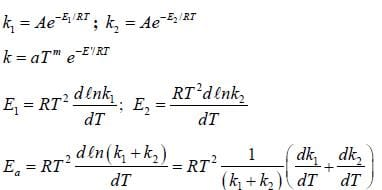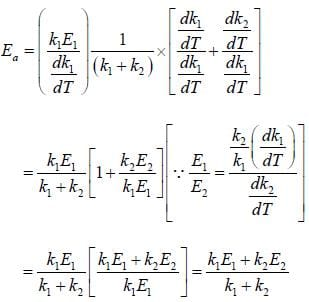Chemical Kinetics - Question 2

### The following reaction was carried out in water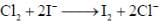the initial concentration of was 0.25 molL–1 and the concentration after 10 min was 0.23 molL–1. The rate of disappears of I– (in untis of M min–1) and rate of appearance of I2​.

Detailed Solution for Chemical Kinetics - Question 2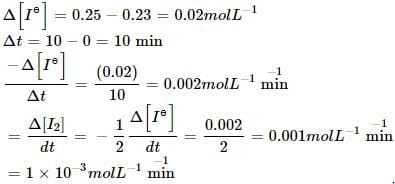Chemical Kinetics - Question 3

### For the reaction, A + B → C +D, Δh = 20 kJ/mole. The activation energy of the forward reaction is 85 kJ/mole. The activation energy of the reverse reaction

Detailed Solution for Chemical Kinetics - Question 3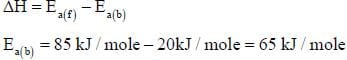*Answer can only contain numeric values
Chemical Kinetics - Question 4

Consider the following variation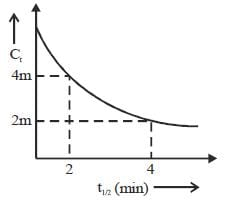The order of reaction is _______________

Detailed Solution for Chemical Kinetics - Question 4

For second order when concentration halved t1/2 become double.

*Answer can only contain numeric values
Chemical Kinetics - Question 5

For the following sequential reaction,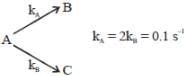time at which [B] maximum is _____(in sec).

Detailed Solution for Chemical Kinetics - Question 5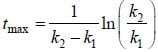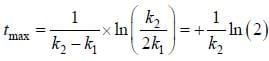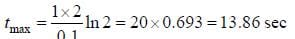*Answer can only contain numeric values
Chemical Kinetics - Question 6

The percent transmittance of a solution having absorbance (optical density) 1.0 is ____________

Detailed Solution for Chemical Kinetics - Question 6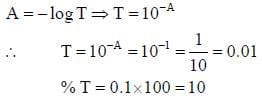Chemical Kinetics - Question 7

Which of the following curves represent(s) a zero order reaction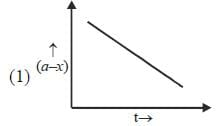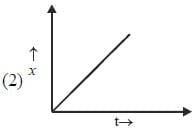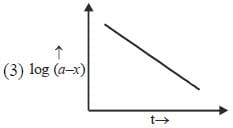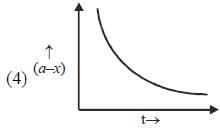Chemical Kinetics - Question 8

A catalyst increases the rate of a chemical reaction by
(1) increasing the activation energy
(2) decreasing the activation energy
(3) increasing the average K.E. of the molecule
(4) increasing the number of active molecules.

Chemical Kinetics - Question 9

The rate of the elementary reaction at 25oC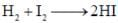Is given by rate = 1.7 x 10-18[H2][I2] - 2.4 x 10-21 [HI]2
The rate of decomposition of gaeseous HI to H2 and I2 at 25ºC is given by the equilibrium constant for the formation of HI from H2 and I2 at 25ºC is

Detailed Solution for Chemical Kinetics - Question 9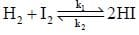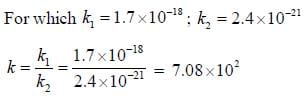Chemical Kinetics - Question 10

Time constant τ is the required for the conc. of a reactant to fall to 1/e of its initial value then?

Detailed Solution for Chemical Kinetics - Question 10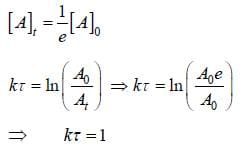Chemical Kinetics - Question 11

For the first order reaction,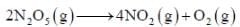A is 4.3 x 1013 s-1 and Ea is 103.35 KJ/mole. The value of k at 300K is

Detailed Solution for Chemical Kinetics - Question 11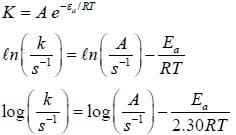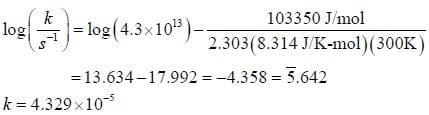Chemical Kinetics - Question 12

The frequency factors for a unimolecular gas reaction occuring at 473K is 2.5×1013 s–1 . The value of entropy of activation is

Detailed Solution for Chemical Kinetics - Question 12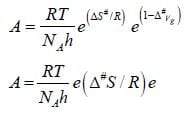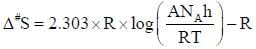Chemical Kinetics - Question 13

Cyclohexane inter converts between a chair and a boat structure. The activation parameters for the reaction from the chair to boat form of the molecule are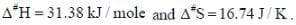The value of standard Gibbs energy of activation and the rate constant and the rate constant for this reaction at 325K

Detailed Solution for Chemical Kinetics - Question 13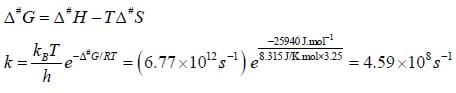Chemical Kinetics - Question 14

The rate law for the reaction between Co(g) to form phosgene (Cl2CO)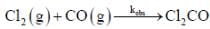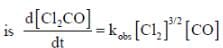Show that the following mechanism is consistent with this rate law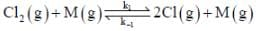(Fast equilibrium)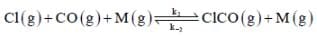(Fast equilibrium)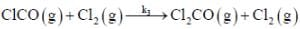(Slow)
Where M is any gas molecule present in the reaction container. The value of kobs in terms of the rate constants for the individual. Steps of the reaction mechanism

Detailed Solution for Chemical Kinetics - Question 14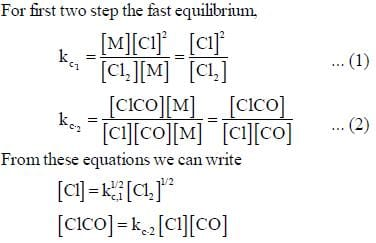Using the third step of the reaction mechanism to write d[CL2CO] / dt and equations  (1) and (2)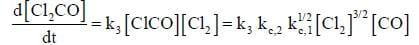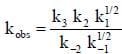*Answer can only contain numeric values
Chemical Kinetics - Question 15

A substance, when dissolved in water at 10-3 M, absorbs 10% of incident radiation in a path of 1 cm length. What should be the concentration of the solution if it were to absorb 90% of the same radiation?

Detailed Solution for Chemical Kinetics - Question 15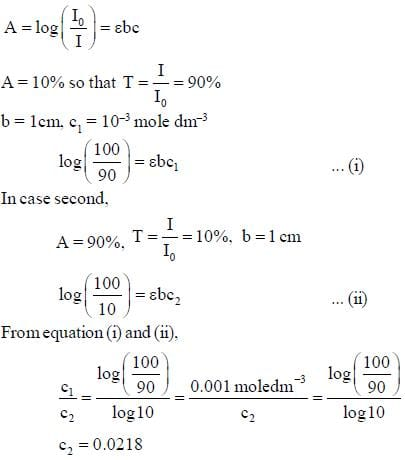*Answer can only contain numeric values
Chemical Kinetics - Question 16

For a reaction with rate equation - dc/dt = kc2, Co and C are the concentration of the reactant at time zero and t respectively. If 10 minutes were required for C0 to becomes C0/2 the time required for Co to becomes C0/4 is ____ min. (Answer should be an integer).

Detailed Solution for Chemical Kinetics - Question 16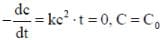On integration by taking time 0 to t, we get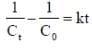...(i)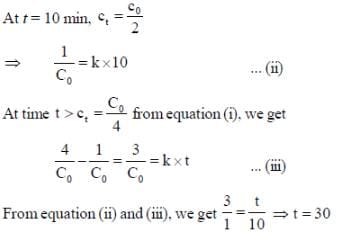*Answer can only contain numeric values
Chemical Kinetics - Question 17

The concentration of non-competitive inhibitor(k1 = 2.9 x 10-4 moldm-3) needed to yield to 90% inhibiten of an enzyme catalysed reaction is ______mol dm-3. (Round off to two decimal places).

Detailed Solution for Chemical Kinetics - Question 17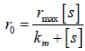...(i)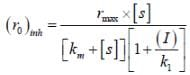...(ii)
(i)/(ii), we get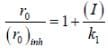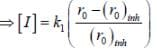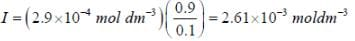*Answer can only contain numeric values
Chemical Kinetics - Question 18

During Nuclear-explosion, one of the product is 90Sr with half-life is 28.1 year. If 1μg od 90Sr was absorbed in the bones of a newly born baby instead of calcium. How much of it will remain after 10 year if it is not lost metabolically? (in unit of μg) (Round off to two decimal places).

Detailed Solution for Chemical Kinetics - Question 18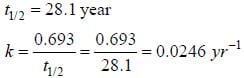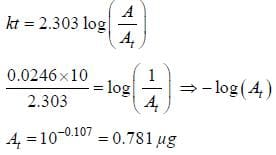*Answer can only contain numeric values
Chemical Kinetics - Question 19

In a homogeneous catalytic reaction, 1.0 M of a substrate band, 1.0 μM of a catalyst yield 1.0 mM of a product in 10S. The turnover frequency (TOF) of the reaction(s-1) is ______(answer should be an integer)

Detailed Solution for Chemical Kinetics - Question 19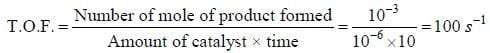Chemical Kinetics - Question 20

In the Linewear Burk plot of (initial rate)–1 vs (initial substrate concentration)–1. For an enzyme catalyzed reacts following Michael Menten mechanism. The y-intercept is 6000 M–1s. If the initial enzyme concentration is 1×10–5 M. The turn over number is

Detailed Solution for Chemical Kinetics - Question 20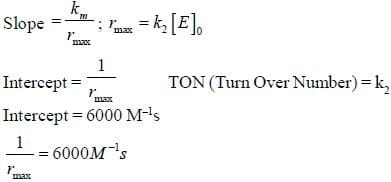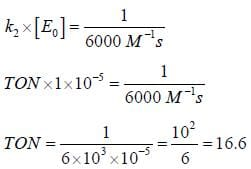## GATE Chemistry Mock Test Series

1 docs|34 tests
 Use Code STAYHOME200 and get INR 200 additional OFF Use Coupon Code